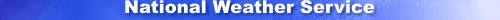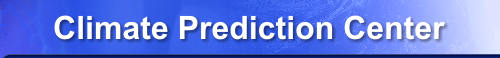Home Site Map News Organization Search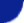CPC Search Related Outlooks    6-10 Day    8-14 Day    Monthly    Seasonal Related Observations    Temperature    Precipitation Related Verifications    6-10 Day / 8-14 Day    Monthly    Seasonal About Us    Our Mission    Who We Are Contact Us    CPC Information    CPC Web Team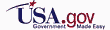## How to use the Verification Web Tool

What is verification?

Verification of forecasts involves comparing the skill of previously made forecasts to what was actually observed. Skill is a relative measure of forecast performance. This tool enables users to select sub-sets of (aggregate) forecasts and perform various statistical calculations to provide objective metrics on the skill of the forecasts.

By allowing users to investigate the skill of forecasts by various aspects, users can have more specific assessments that can help them decide how to use real-time forecasts. For example, decision makers can inspect the skill score results for various situations that can help them assess their confidence in using real-time forecasts in similar situations.

[+] Heidke

The Heidke Skill Score (HSS) compares how often the forecast category correctly match the observed category, over and above the number of correct "hits" expected by chance alone.

This score utilizes the number of correct and incorrect category hits. The values range from -50 to 100. A score of 100 indicates a perfect forecast and a score of -50 indicates a perfectly incorrect forecast. Scores greater than 0 indicate improvement compared to a random forecast and indicate skill.

For monthly and seasonal forecasts, the equal chances (EC) forecast category are included in scores.

The equation for the score is:

`HSS (%) = 100 * (H - E) / (T - E)`

where H = Number of correct forecasts, E = Expected number of correct forecasts (1/3 of total), and T = Total number of valid forecast-observation pairs.

The equation for the score including EC forecasts is:

`HSSwithEC (%) = HSS * coverage`

where coverage = number of non EC forecasts/Total.

[+] RPSS

The Rank Probability Skill Score (RPSS) measures the improvement of multi-category forecasts relative to a reference forecast and takes into account the probability for each category.

Ranked Probability Score (RPS) is a squared error score with respect to the cumulative probabilities for multi-category forecasts and whether or not the event occurred. The Ranked Probability Skill Score (RPSS) measures the improvement of the multi-category forecast relative to a reference forecast (the sample climatology). RPSS values range from -Infinity to 1. Forecasts made with higher probabilities are penalized heavily if they are wrong, and forecasts made with lower probabilities are penalized less severely if they are wrong, since they aren't expected to be correct as often. Conversely, forecasts made with higher probabilities are rewarded more heavily if they are correct, and forecasts made with lower probabilities are rewarded less heavily if they are correct.

EC forecasts for monthly and seasonal forecasts are included in scores.

The equation for the score is:

`RPSS = 1 - RPSforecast/RPSreference`

The RPS score representing a collection of forecasts in space or time is: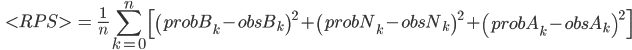where n is the total number of valid forecast-observation pairs. For the chart, each forecast data point in space is evaluated and represents each kth value. probBk, probNk, probAk are the probabilities of the below normal, near normal, and above normal forecast categories respectively at each point k, and obsBk, obsNk, obsAk are either 0 if that category was observed, or 1 if that category was not observed at each point k.

The score is the sum of the squares of the difference between the forecast probability of each category and either "0" or "1" for the category observed at that point over all forecast points, divided by the total number of forecast points used. To get RPSforecast, the forecast probabilities are used. To get RPSreference, cumulative probabilities starting at 1/3 are used for each category.

To learn about what each of the options, you can click on the question mark next to each option label to pop-out more information about each.

In general, the difference between the "Chart" and "Map" tabs is that the chart displays time series of scores. These scores represent the Continental US (CONUS) at each time step. The map represents scores for each location across the period of time displayed in the titles of the graphics.

[+] Interpreting Charts

The labels on the x-axis of the charts represent the center dates of the valid periods. The term 'valid period' refers to the period that the forecast was made to represent. The best way to think of this is to think of the chart results in terms of "how well the forecast of a range of time (which is represented by the center date) compared to what was actually observed".

Even though the date in the time series chart results may be labeled as a single day, it does not mean that it represents a daily forecast. The 6-10 day and 8-14 day forecasts represent a range of time across 5 days and 7 days, respectively. Each day, the Climate Prediction Center releases updated forecast maps that represent these time periods. In the verification results, 6-10 day and 8-14 day forecasts are labeled as "YYYYMMDD", which is the center day in the time range the forecast is for.

For example, a score value on the resulting time series chart with the date 7/30/2011 for a 6-10 day forecast (which spans across 5 days), represents the skill of the forecast that was made for the period July 28, 2011 - August 1, 2011. If this date is shown for an 8-14 day forecast (which spans across 7 days), the skill score represents the skill of the forecast made for July 27, 2011 - August 2, 2011. In both cases, July 30, 2011 is the middle date of those periods.

Additionally, a chart date can have the format "YYYYMM". If this applies to a monthly forecast skill score, the value is for that month and year. However, if it is a seasonal forecast score, the date on the results represents the middle month and year of the 3-month period that the forecast is valid for. For example, a score value for 7/2011 represents a seasonal forecast valid for June-July-August 2011.

The y-axis represents the score values.

All the forecast and observation datasets used to produce the scores are in a 2 x 2 degree gridded format originally.

The categories of observations are used in the skill scores along with the forecast categories. The observation categories are determined by using the climatology data from 1981-2010 as the reference period.

[+] Warnings and Errors

Sometimes text such as "Image not available" may be displayed instead of some graphics on the page. To get more details on why a graphic is not displayed, you can click the "Why?" link where the graphic should be, which will jump to the warnings and errors section at the bottom of the page.

You can download or view data in ASCII text format by using the 'Download data' links under the associated graphics. The header of the file contains information about the options used in the score calculations and uses a pound '#' symbol at the beginning of each header line. The percent and number of valid scores are included in the header. This represents the percent and number of scores in the file that are considered valid (non-NaN values).

The data in the ASCII files is space delimited. Missing values are denoted as 'NaN'.

The content and header names (described below in single quotes and bold font) of the columns are described below in the order they are listed in the file from the left to right:

• Reference point - The type of data in this column depends on whether it represents a chart or map :
• chart ('Date_Valid') : Valid date of forecast. The date is expressed as the middle date of the valid period that the forecast represents. This is formatted as either MM/dd/yyyy (medium-range) or MM/yyyy (long-range).
• map ('id') : Assigned gridpoint value formatted as XXYY, where XX and YY are zero-padded integers representing a gridpoint in the X and Y location, respectively. The bottom left gridpoint is 0101 and the top right gridpoint is 3619.
• Latitude and longitude ('lat','lon') - This is for maps only. Position of the associated gridpoint location. The lat and lon represents the center point of the associated gridpoint.
• Forecast source ('Forecast_Source') - Forecast type (ie. 'manual' which is the official CPC forecast).
• Scores ('all_cats')- Skill scores. The header is labeled 'all_cats' because the scores take into account all forecast categories (above-normal, normal, and below-normal) in the score calculation.
• Percent Valid Dates ('Percent_Valid_Locations','Percent_Valid_Dates')- Percent valid (non-NaN) locations (dates) that went into the calculation of each score for charts (maps).

[+] Warnings and Errors

Sometimes text such as "Image not available" may be displayed instead of some graphics on the page. To get more details on why a graphic is not displayed, you can click the "Why?" link where the graphic should be, which will jump to the warnings and errors section at the bottom of the page.